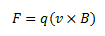# When a charged particle moves perpendicular to a magnetic field, then (1) Speed of the particle is changed. (2) Speed of the particle remains unchanged. (3) Direction of the particle remains unchanged. (4) Acceleration of the particle remains unchanged.

Answer:(2) Speed of the particle remains unchanged.

A force acting on a particle is said to perform work when there is a component of the force in the direction of motion of the particle.

• In the case under consideration where we have a charged particle carrying a charge q moving in a uniform magnetic field of magnitude B, the magnetic force acts perpendicular to the velocity of the particle.
• Here we say that no work is done by the magnetic force on the particle and hence, no change in the velocity of the particle can be seen.

Mathematically, when the velocity of the particle v is perpendicular to the direction of the magnetic field, we can writeWhen the particle’s velocity is perpendicular to the magnetic field, the magnetic force is perpendicular to both, and the particle moves in a circle at constant speed v.(1)(0)